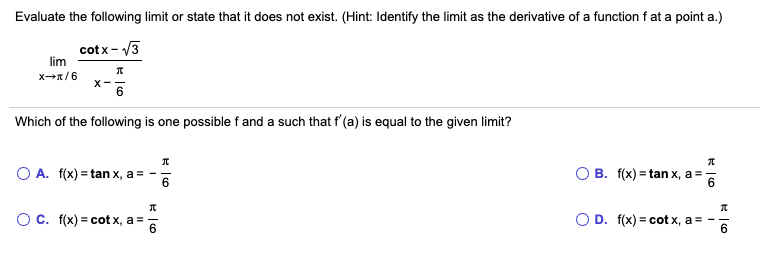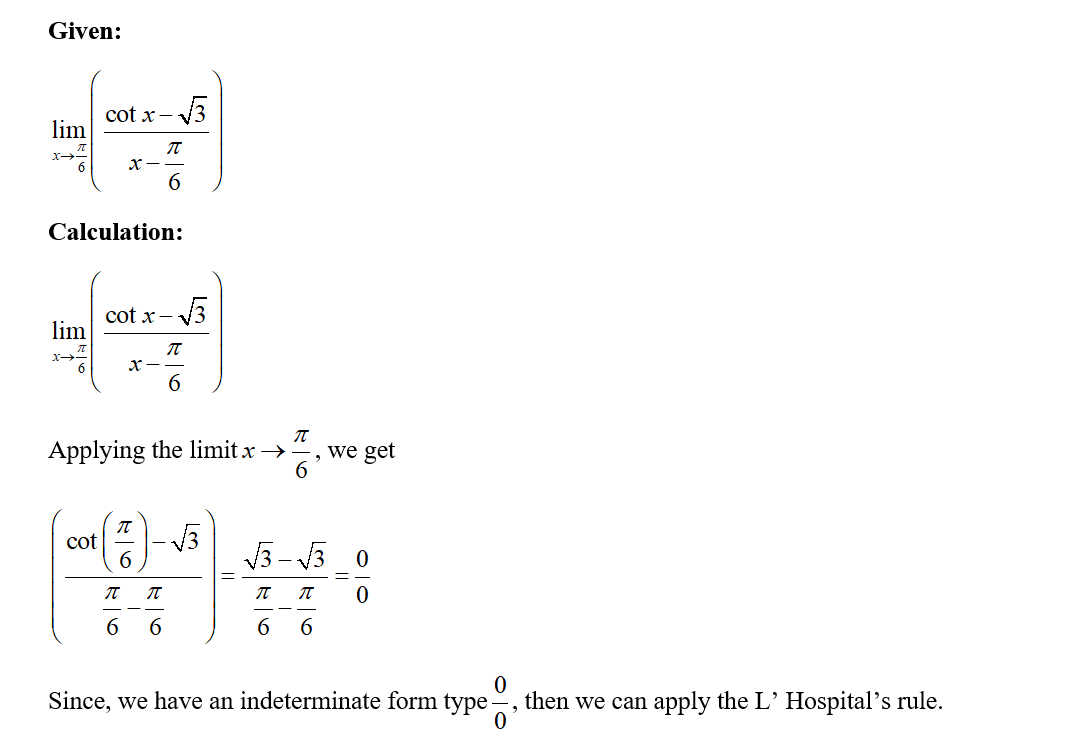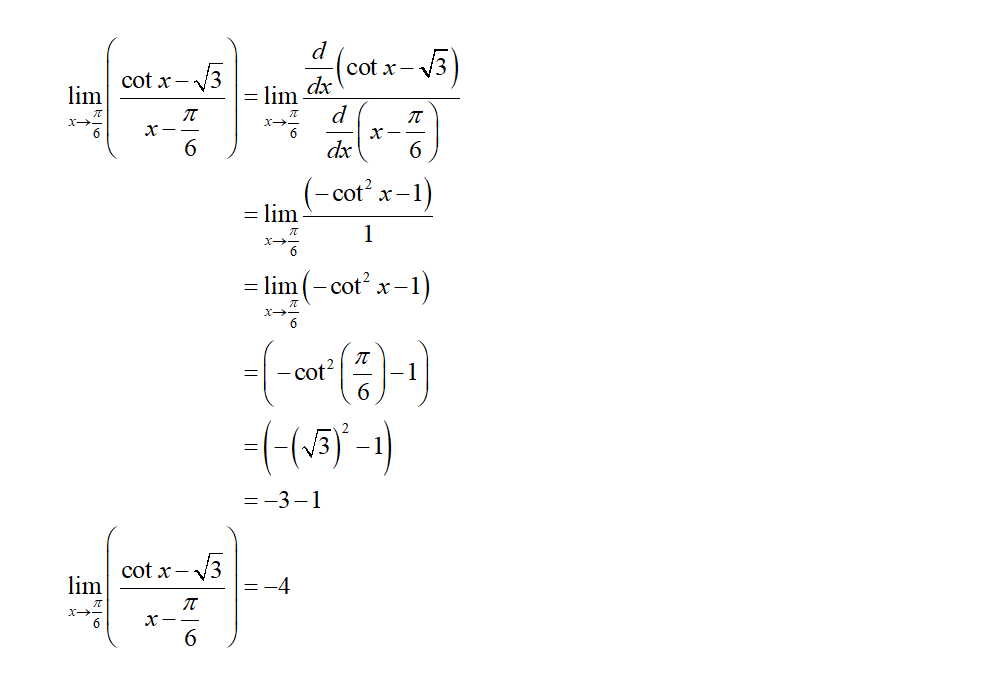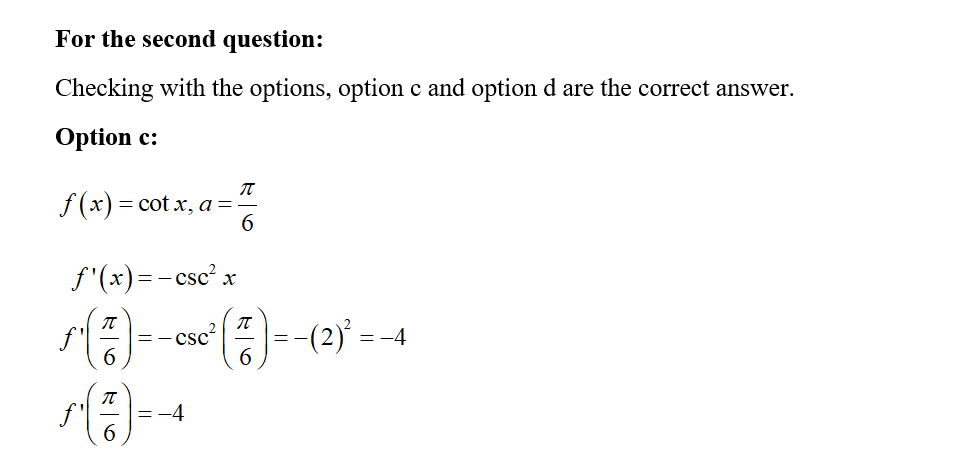# Evaluate the following limit or state that it does not exist. (Hint: Identify the limit as the derivative of a function f at a point a.)limcotx- V3X-Which of the following is one possible f and a such that f'(a) is equal to the given limit?O A. f(x) = tan x, a = -O B. f(x) = tan x, a =6.O c. f(x) = cot x, a =O D. f(x) = cot x, a6.

Question
8 viewshelp_outlineImage TranscriptioncloseEvaluate the following limit or state that it does not exist. (Hint: Identify the limit as the derivative of a function f at a point a.) lim cotx- V3 X- Which of the following is one possible f and a such that f'(a) is equal to the given limit? O A. f(x) = tan x, a = - O B. f(x) = tan x, a = 6. O c. f(x) = cot x, a = O D. f(x) = cot x, a 6. fullscreen
check_circle

Step 1Step 2Step 3...

### Want to see the full answer?

See Solution

#### Want to see this answer and more?

Solutions are written by subject experts who are available 24/7. Questions are typically answered within 1 hour.*

See Solution
*Response times may vary by subject and question.
Tagged in